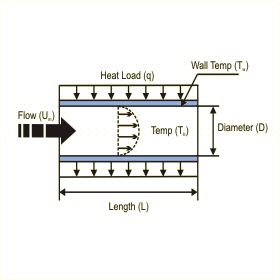Close

This page calculates the heat transfer coefficient for fully developed flow in a smooth circular tube. The convection calculation automatically switches between laminar and turbulent convection correlations based on Reynolds number.The calculation is based on Nusselt number correlations.

The tube wall temperature (Tw) is calculated as:

Tw = Tb + q / (h*A)

Where h is the average heat transfer coefficient, A is the surface area of the tube, q is the heat load uniformly distributed on the tube surface and Tb is the fluid bulk temperature. h is defined as:

h = Nu k / D

Where Nu is the Nusselt Number, k the conductivity of the fluid and D the diameter of the tube. The Nusselt number is calculated as:

For Laminar Flow - Re < 2300
Nu = 4.36 for uniform surface heat flux conditions
friction f = 64 / Re

For Turbulent Flow - 2300 < Re < 5 x 106
Nu = ( f / 8) ( Re - 1000 ) Pr / { 1 + 12.7( f / 8 )1/2 (  Pr2/3 - 1 ) }
where f = ( 0.79ln Re - 1.64 ) -2

Where Re is the Reynolds number and Pr is the Prandtl number are calculated using fluid properties as follows:

Re = fluid density x fluid velocity x diameter / dynamic viscosity
Pr = Specific heat x dynamic viscosity / thermal conductivity

In addition, you must define the fluid properties at the film temperature Tf defined as follows:

Tf = (Tw + Tb) / 2

The above correlations are valid for Prandlt numbers in the range of 0.5 and 2000.

References

Gnielinski, V., Int. Chem. Eng., 16, 359, 1976

Incropera, De Witt., Fundamentals of Heat and Mass Transfer, 3rd ed., John Wiley & Sons, eq.8.53, 8.63a & 8.63b, 1990.